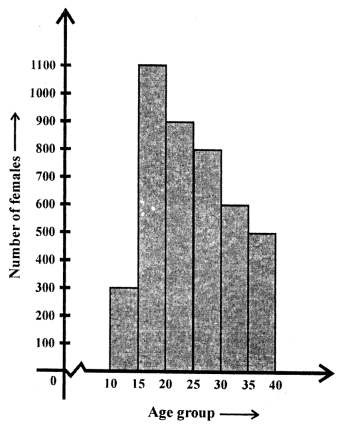## ML Aggarwal Class 8 Solutions for ICSE Maths Chapter 19 Data Handling Ex 19.1

Question 1.
The result of a survey of 200 people about their favourite fruit is given below:Represent the above data by a bar graph.
Solution: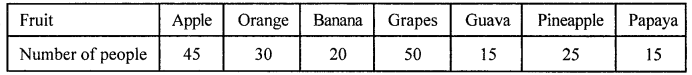The bar graph is given below: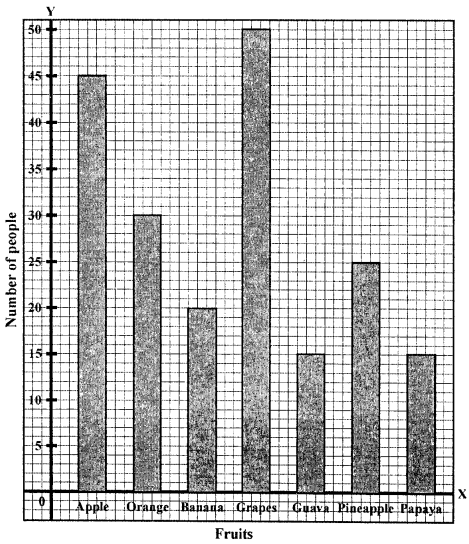Question 2.
Mr Khurana has two kitchen appliance stores. He compares the sales of two stores during a month and recovered as given below:Represent the above data by a double bar graph.
Solution:The double graph of the above given data is given below:Question 3.
The number of goals scored by a football team in different matches is given below:
3, 1, 0, 4, 6, 0, 0, 1, 1, 2, 2, 3, 5, 1, 2, 0, 1, 0, 2, 3, 9, 2, 0, 1, 0, 1, 4, 1, 0, 2, 5, 1, 2, 2, 3, 1, 0, 0, 0, 1, 1, 0, 2, 3, 0, 1, 5, 2, 0
Make a frequency distribution table using tally marks.
Solution:
Frequency table is given below: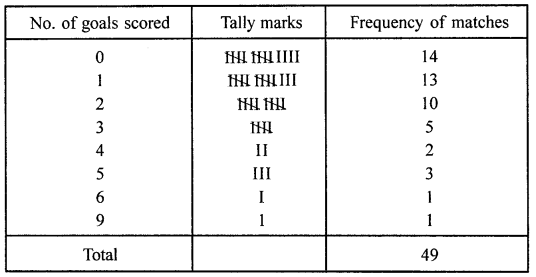Question 4.
Given below a bar graph: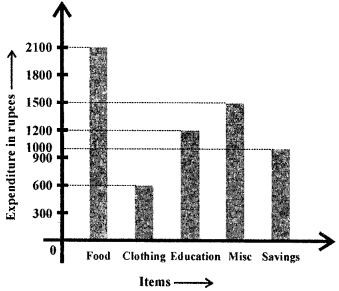(i) What is the information given by the bar graph?
(ii) On which item the expenditure is maximum?
(iii) On which item the expenditure is minimum?
(iv) State whether true or false:
Expenditure on education is twice the expenditure on clothing.
Solution:
(i) In the given bar graph, representation of the expenditure
of monthly salary on different heads is given.
(ii) On food, expenditure is maximum.
(iii) On clothing expenditure is minimum,
(iv) Yes, it is true.

Question 5.
Given below a double bar graph.(i) What is the information given by the double graph?
(ii) Which mode of transport girls using more than the boys?
(iii) Which mode of transport boys using the most?
(iv) In which mode of transport number of girls is half the number of boys?
Solution:
From the double bar graph:
(i) It represent the number of boys and girls using
different modes of transport for going to school.
(ii) In school bus, girls use more than the boys.
(iii) Boy are using the bicycle most.
(iv) In walking, number of girls is half of the number of boys.

Question 6.
Using class intervals 0-5, 5-10, construct the frequency distribution table for the following
data:
13, 6, 12, 9, 11, 14, 2, 8, 18, 16, 9, 13, 17, 11, 19, 6, 7, 12, 22, 21, 18, 1, 8, 12, 18.
Solution:
The required frequency table is given below: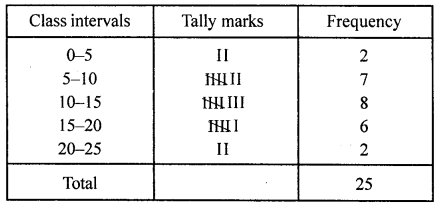Question 7.
Given below are the marks secured by 35 students in a surprise test:
41, 32, 35, 21, 11, 47, 42, 00, 05, 18, 25, 24, 29, 38, 30, 04, 14, 24, 34, 44, 48, 33, 36, 38, 41, 48, 08, 34, 39, 11, 13, 27, 26, 43, 03.
Taking class invervals 0-10, 10-20, …….. construct frequency distribution table. Find the number
of students obtaining below 20 marks.
Solution:
Below is given the frequency table of the given data:Number of students getting below 20 marks are = 5 + 5 = 10

Question 8.
The electricity bills (in ?) of 40 houses in a locality are given below:
78, 87,81,52, 59, 65, 101, 108, 115, 95, 98, 65,62, 121, 128, 63,76, 84, 89,91,65, 101,95,81, 87, 105, 129, 92, 75, 105, 78, 72, 107, 116, 127, 100, 80, 82, 61, 118 Construct a grouped frequency distribution table of class size 10.
Class intervals (Electricity bill in ?) Tally marks Frequency (Number of houses)
Solution: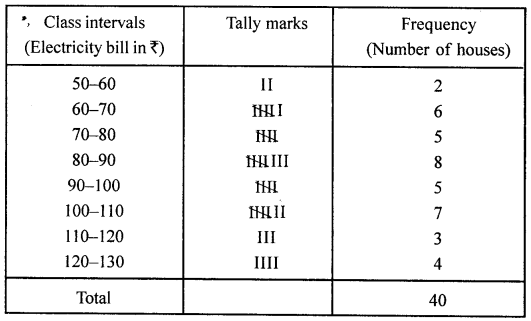Question 9.
Draw a histrogram for the frequency table made for data in Question 8, and answer the following questions:
(i) Which group has the maximum number of houses?
(ii) How many houses pay less than ₹ 100?
(iii) How many houses pay ₹ 100 or more?
Solution:
Histogram of the given data in Question 8, is given below (next page):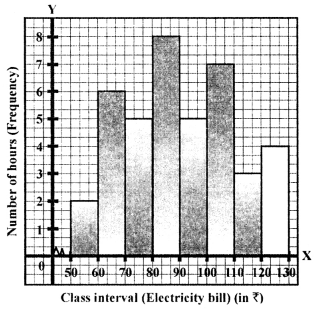(i) Maximum number of house are in the group 80-90.
(ii) Number of houses who play less then ₹100 = 2 + 6 + 5 + 8 + 5 = 26
(iii) Number of houses who pay ₹ 100 or more = 7 + 3 + 4= 14

Question 10.
The weights of 29 patients in a hospital were recorded as follows:Draw a histogram to represent this data visually.
Solution:The histogram of the above-given data is given below: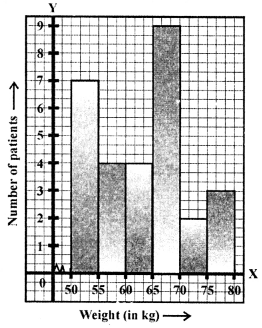Question 11.
In a study of diabetic patients, the following data was obtained: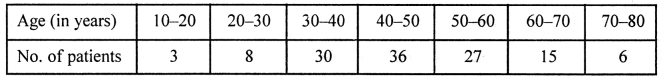Represent the above data by a histogram.
Solution: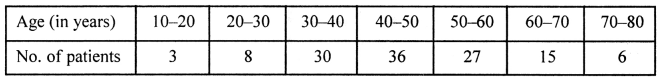The histogram representing the above-given data is given below:Question 12.
The histogram showing the weekly wages (in ₹) of workers in a factory is given alongside: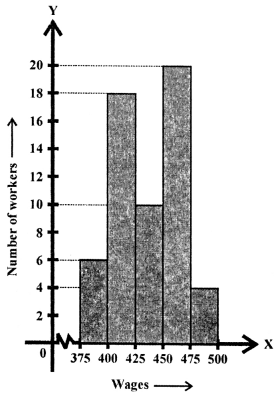(i) What is the frequency of class 400-425?
(ii) What is the class having a minimum frequency?
(iii) How many workers get more than ₹425?
(iv) How many workers get less than ₹475?
(v) Number of workers whose weekly wages are more than or equal to ₹400 but less than ₹450.
Solution:
In the given histogram showing the weekly wages of workers in a factory.
(i) Frequency of class 400-425 is 18.
(ii) Class 475-500 has the minimum frequency.
(iii) Number of workers getting more then ₹425 is 34.
(iv) Number of workers getting less than ₹475 is 54.
(v) Number of workers whose weekly wages is more than
or equal to ₹400 but less than ₹450 = 28.

Question 13.
The number of hours for which students of a particular class watched television during holidays is shown in the histogram below.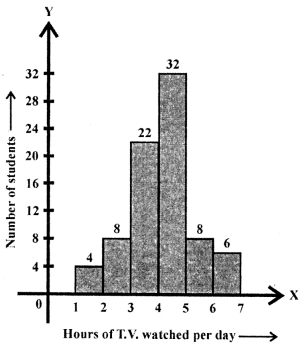(i) For how many hours did the maximum number of students watch T.V.?
(ii) How many students watched T.V. for less than 4 hours?
(iii) How many students spent more than 5 hours in watching T.V.?
(iv) How many students spent more than 2 hours but less than 4 hours in watching T.V.?
Solution:
From the histogram given,
(i) Maximum number of students who watch 4-5 hours.
(ii) Number of students who watch T.V. for less than 4 hours is 34.
(iii) Number of students who spent more than 5 hours in watching the T.V. is 14.
(iv) Number of students who spent more than 2 hours
but less than 4 hours watching T.V. is 30.

Question 14.
The number of literate females in the age group of 10 to 40 years in a town is shown in the histogram alongside.